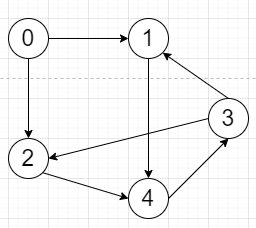# Program to calculate vertex-to-vertex reachablity matrix in Python

Suppose we have a graph as an adjacency list representation, we have to find 2D matrix M where

• M[i, j] = 1 when there is a path between vertices i and j.

• M[i, j] = 0 otherwise.

So, if the input is likethen the output will be

 1 1 1 1 1 0 1 1 1 1 0 1 1 1 1 0 1 1 1 1 0 1 1 1 1

To solve this, we will follow these steps −

• ans:= a 2d matrix of size n x n, where n is the number of vertices, fill with 0s

• for i in range 0 to n, do

• q:= a queue, and insert i at first

• while q is not empty, do

• node:= first element of q, and delete first element from q

• if ans[i, node] is non-zero, then

• go for next iteration

• ans[i, node]:= 1

• neighbors:= graph[node]

• for each n in neighbors, do

• insert n at the end of q

• return ans

Let us see the following implementation to get better understanding −

## Example

class Solution:
def solve(self, graph):
ans=[[0 for _ in graph] for _ in graph]
for i in range(len(graph)):
q=[i]
while q:
node=q.pop(0)
if ans[i][node]: continue
ans[i][node]=1
neighbors=graph[node]
for n in neighbors:
q.append(n)
return ans
ob = Solution()
priunt(ob.solve(adj_list))

## Input

[[1,2],,,[1,2],]

## Output

[[1, 1, 1, 1, 1],
[0, 1, 1, 1, 1],
[0, 1, 1, 1, 1],
[0, 1, 1, 1, 1],
[0, 1, 1, 1, 1]
]